# Cryptography Block Ciphers David Brumely Carnegie Mellon University

• Slides: 55Cryptography: Block Ciphers David Brumely Carnegie Mellon University Credits: Slides originally designed by David Brumley. Many other slides are from Dan Boneh’s June 2012 Coursera crypto class.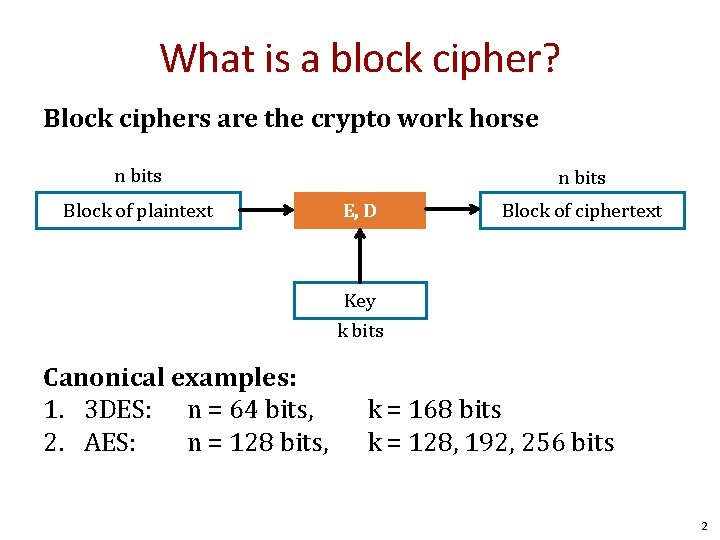What is a block cipher? Block ciphers are the crypto work horse n bits Block of plaintext n bits E, D Block of ciphertext Key k bits Canonical examples: 1. 3 DES: n = 64 bits, 2. AES: n = 128 bits, k = 168 bits k = 128, 192, 256 bits 2Stream Ciphers Recall: A stream cipher typically xors plaintext byte-by-byte with PRNG(k) Example: RC 4 (Rivest Cipher 4) is a PRNG based on a key, and is used as a stream cipher in TLS and WPA This differs from a block cipher where we operate on blocks of plaintext, not byte-by-byte in a streaming fashion. 3Block ciphers built by iteration key k key expansion key k 1 m m R(k 1, ∙) key k 2 m 1 R(k 2, ∙) key k 3 m 2 R(k 3, ∙) key kn m 3 R(kn, ∙) R(k, m) is called a round function invoked up to n times Ex: 3 DES (n=48), AES 128 (n=10) c 4Performance: Stream vs. block ciphers Crypto++ 5. 6. 0 [Wei Dai] AMD Opteron, 2. 2 GHz (Linux) Stream Cipher Block/key size Throughput [MB/s] RC 4 126 Salsa 20/12 643 Sosemanuk 727 Block 3 DES AES 128 64/168 128/128 13 109 5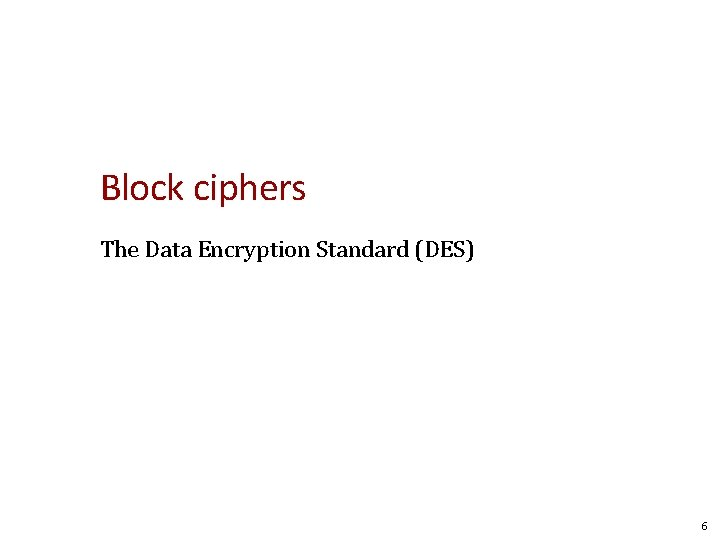Block ciphers The Data Encryption Standard (DES) 6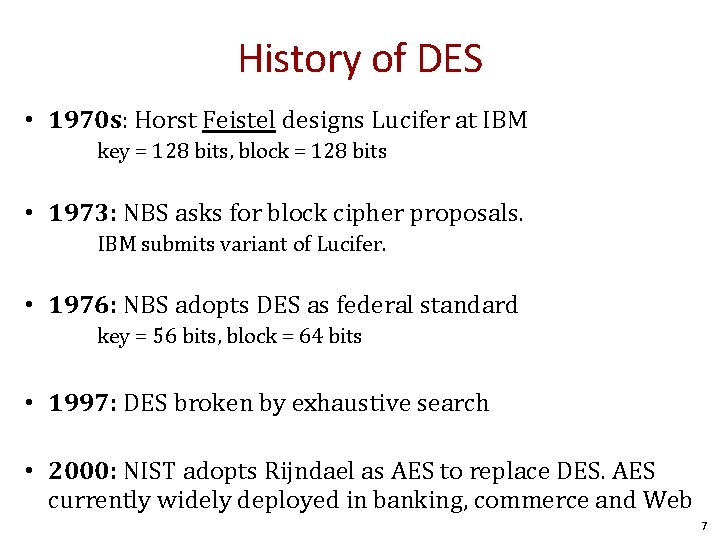History of DES • 1970 s: Horst Feistel designs Lucifer at IBM key = 128 bits, block = 128 bits • 1973: NBS asks for block cipher proposals. IBM submits variant of Lucifer. • 1976: NBS adopts DES as federal standard key = 56 bits, block = 64 bits • 1997: DES broken by exhaustive search • 2000: NIST adopts Rijndael as AES to replace DES. AES currently widely deployed in banking, commerce and Web 7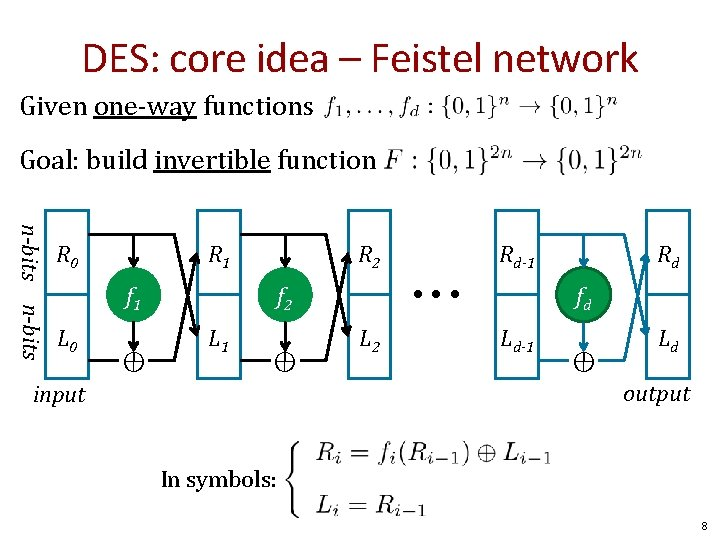DES: core idea – Feistel network Given one-way functions Goal: build invertible function n-bits R 0 R 1 R 2 f 1 L 0 ⊕ • • • f 2 L 1 ⊕ Rd-1 L 2 Rd fd Ld-1 ⊕ Ld output input In symbols: 8Feistel network - inverse Claim: Feistel function F is invertible Proof: construct inverse Ri+1 fi+1 Li ⊕ ⊕ Ri Ri fi+1 Li+1 Li 9Decryption circuit ⊕ n-bits fd Ld ⊕ Rd-1 Rd-2 • • • fd-1 Ld-1 R 1 Ld-2 ⊕ n-bits Rd R 0 f 1 L 0 • Inversion is basically the same circuit, with f 1, …, fd applied in reverse order • General method for building invertible functions (block ciphers) from arbitrary functions. • Used in many block ciphers … but not AES 10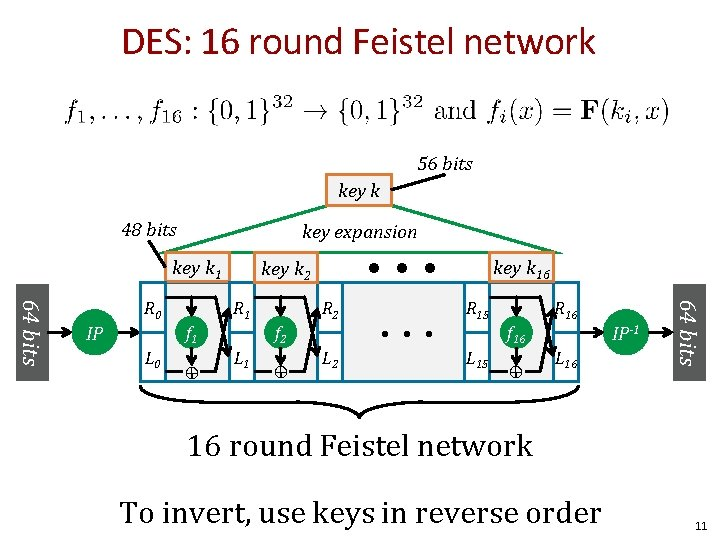DES: 16 round Feistel network 56 bits key k 48 bits key expansion key k 1 f 2 f 1 L 0 ⊕ R 2 L 1 ⊕ L 2 • • • key k 16 R 15 f 16 L 15 ⊕ L 16 IP-1 64 bits IP R 1 R 0 • • • key k 2 16 round Feistel network To invert, use keys in reverse order 11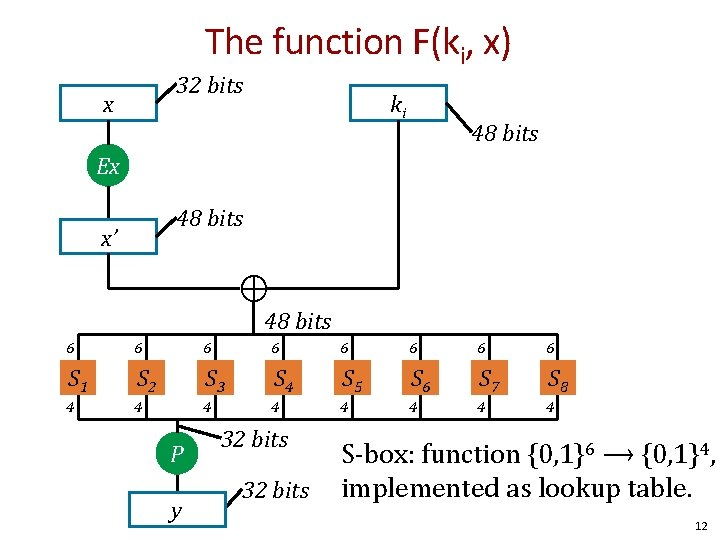The function F(ki, x) 32 bits x ki 48 bits Ex 48 bits x’ ⊕ 48 bits 6 6 6 6 S 1 S 2 S 3 S 4 S 5 S 6 S 7 S 8 4 4 4 4 P y 32 bits S-box: function {0, 1}6 ⟶ {0, 1}4, implemented as lookup table. 12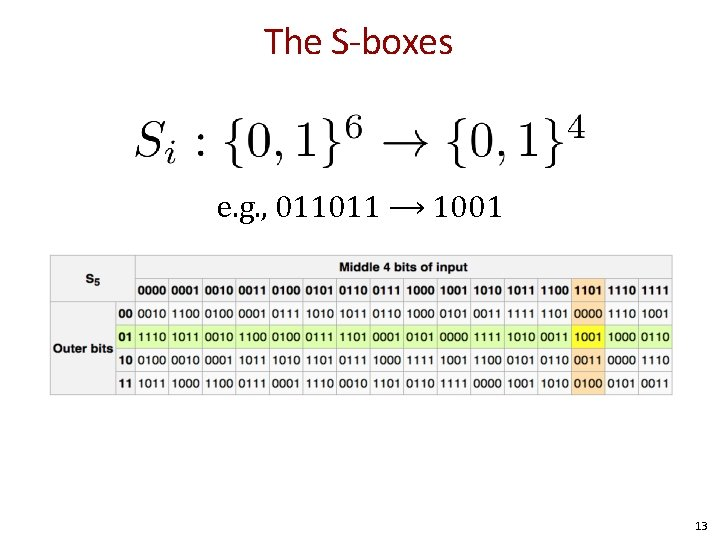The S-boxes e. g. , 011011 ⟶ 1001 13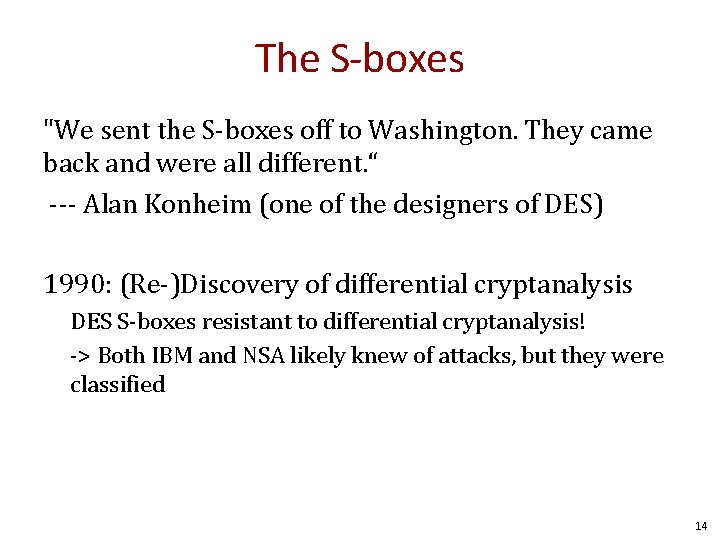The S-boxes "We sent the S-boxes off to Washington. They came back and were all different. “ --- Alan Konheim (one of the designers of DES) 1990: (Re-)Discovery of differential cryptanalysis DES S-boxes resistant to differential cryptanalysis! -> Both IBM and NSA likely knew of attacks, but they were classified 14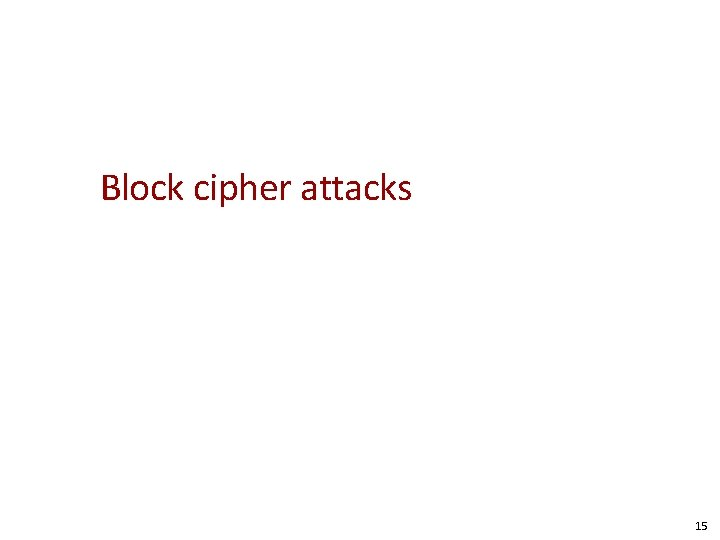Block cipher attacks 15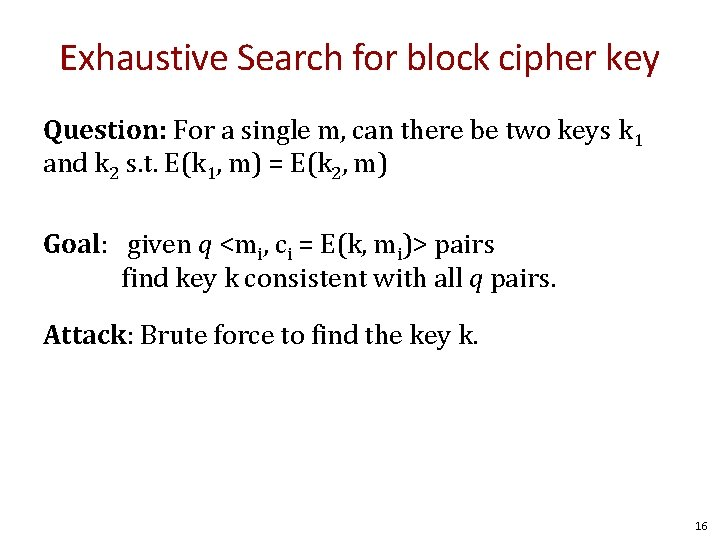Exhaustive Search for block cipher key Question: For a single m, can there be two keys k 1 and k 2 s. t. E(k 1, m) = E(k 2, m) Goal: given q <mi, ci = E(k, mi)> pairs find key k consistent with all q pairs. Attack: Brute force to find the key k. 16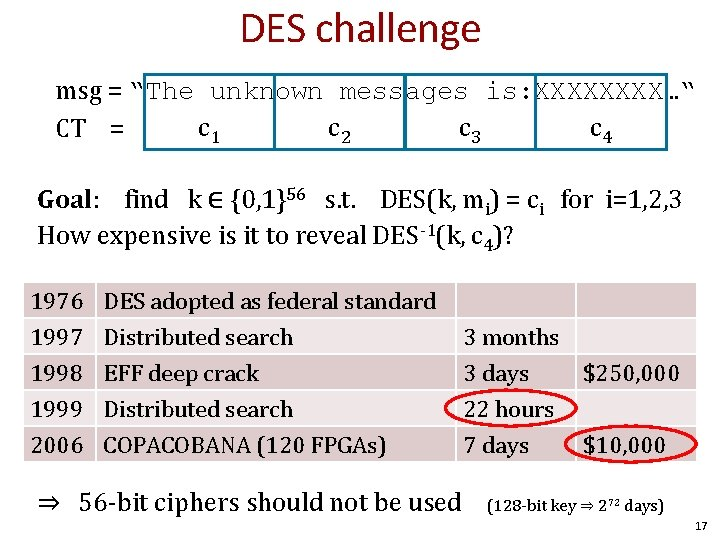DES challenge msg = “The unknown messages is: XXXX…“ c 1 c 2 c 3 c 4 CT = Goal: find k ∈ {0, 1}56 s. t. DES(k, mi) = ci for i=1, 2, 3 How expensive is it to reveal DES-1(k, c 4)? 1976 1997 1998 1999 DES adopted as federal standard Distributed search EFF deep crack Distributed search 2006 COPACOBANA (120 FPGAs) ⇒ 56 -bit ciphers should not be used 3 months 3 days \$250, 000 22 hours 7 days \$10, 000 (128 -bit key ⇒ 272 days) 17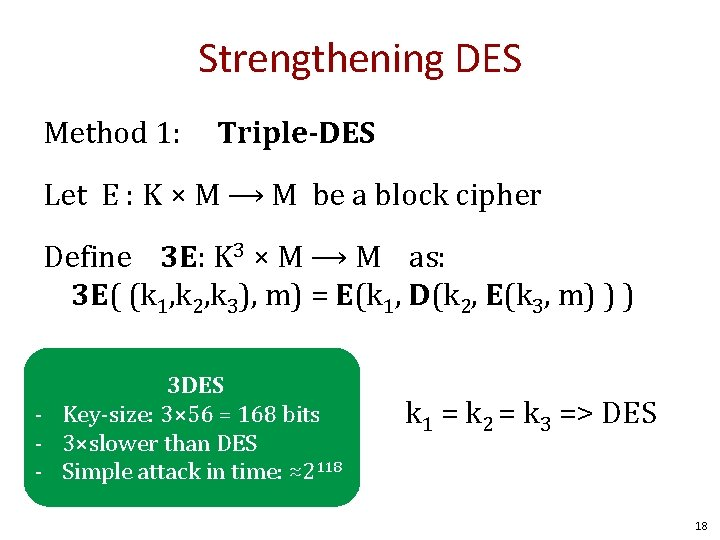Strengthening DES Method 1: Triple-DES Let E : K × M ⟶ M be a block cipher Define 3 E: K 3 × M ⟶ M as: 3 E( (k 1, k 2, k 3), m) = E(k 1, D(k 2, E(k 3, m) ) ) 3 DES - Key-size: 3× 56 = 168 bits - 3×slower than DES - Simple attack in time: ≈2118 k 1 = k 2 = k 3 => DES 18Why not 2 DES? 1. Meet in the Middle Attacks 2. Time to break cipher analysis 19Why not 2 DES? • Define 2 E( (k 1, k 2), m) = E(k 1 , E(k 2 , m) ) key-len = 112 bits for 2 DES m E(k 2, ⋅) E(k 1, ⋅) c Given: M = (m 1, …, m 10), C = (c 1, …, c 10). (Naïve method) For each k 2∈{0, 1}56: For each k 1∈{0, 1}56: if E(k 1, E(k 2, mi)) = ci then (k 2, k 1) k 2 m … c' … k 1 … c’’ … 2112 checks c’’ = c? 20Meet in the middle attack • Define 2 E( (k 1, k 2), m) = E(k 1 , E(k 2 , m) ) key-len = 112 bits for 2 DES m E(k 2, ⋅) E(k 1, ⋅) … m c' … c’’ c … Idea: key found when c’ = c’’: E(ki, m) = D(kj, c) 21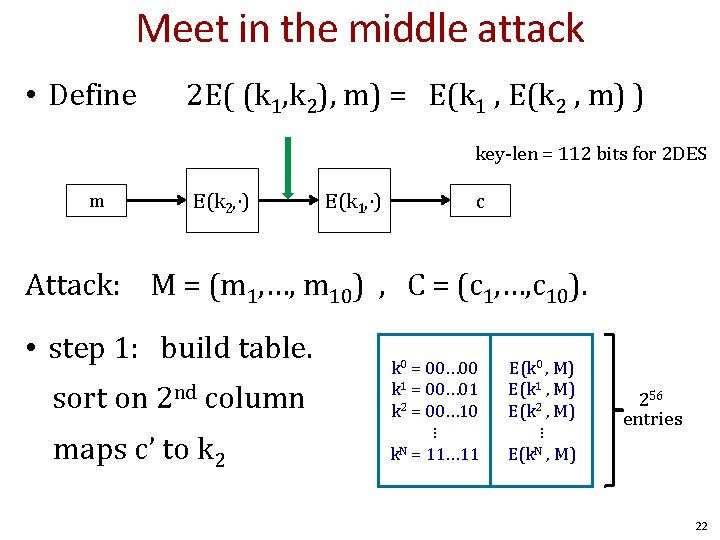Meet in the middle attack • Define 2 E( (k 1, k 2), m) = E(k 1 , E(k 2 , m) ) key-len = 112 bits for 2 DES m E(k 2, ⋅) E(k 1, ⋅) c Attack: M = (m 1, …, m 10) , C = (c 1, …, c 10). • step 1: build table. sort on 2 nd column maps c’ to k 2 k 0 = 00… 00 k 1 = 00… 01 k 2 = 00… 10 ⋮ k. N = 11… 11 E(k 0 , M) E(k 1 , M) E(k 2 , M) ⋮ E(k. N , M) 256 entries 22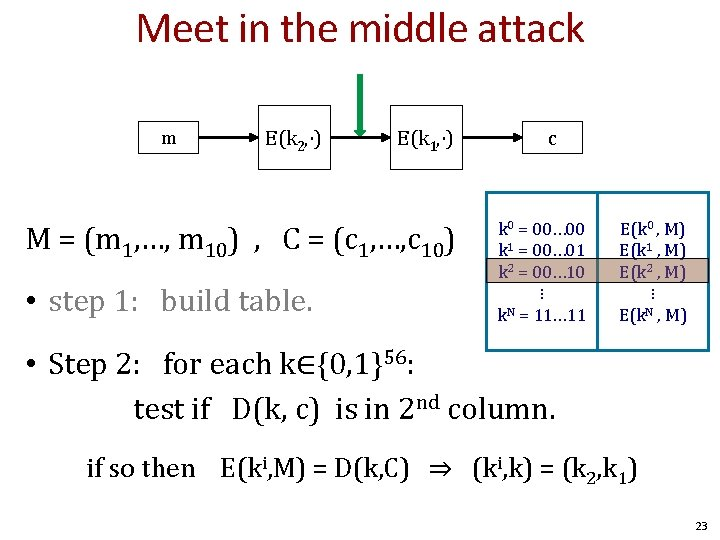Meet in the middle attack m E(k 2, ⋅) E(k 1, ⋅) M = (m 1, …, m 10) , C = (c 1, …, c 10) • step 1: build table. c k 0 = 00… 00 k 1 = 00… 01 k 2 = 00… 10 ⋮ k. N = 11… 11 E(k 0 , M) E(k 1 , M) E(k 2 , M) ⋮ E(k. N , M) • Step 2: for each k∈{0, 1}56: test if D(k, c) is in 2 nd column. if so then E(ki, M) = D(k, C) ⇒ (ki, k) = (k 2, k 1) 23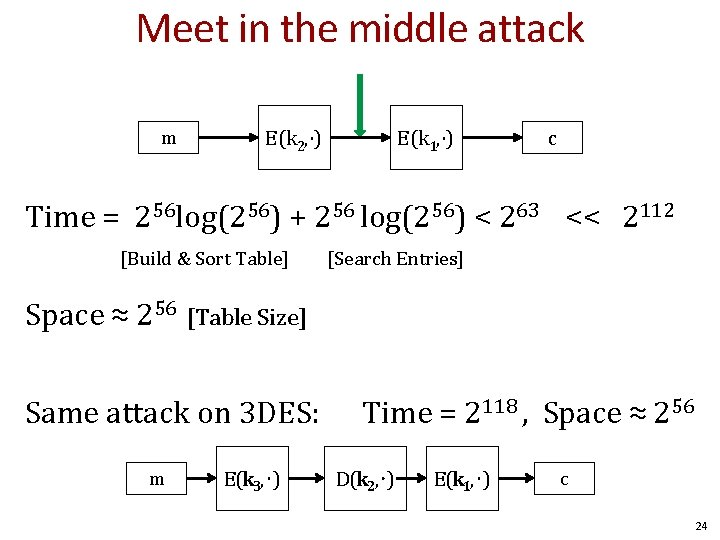Meet in the middle attack m E(k 2, ⋅) E(k 1, ⋅) c Time = 256 log(256) + 256 log(256) < 263 << 2112 [Build & Sort Table] [Search Entries] Space ≈ 256 [Table Size] Same attack on 3 DES: m E(k 3, ⋅) Time = 2118 , Space ≈ 256 D(k 2, ⋅) E(k 1, ⋅) c 24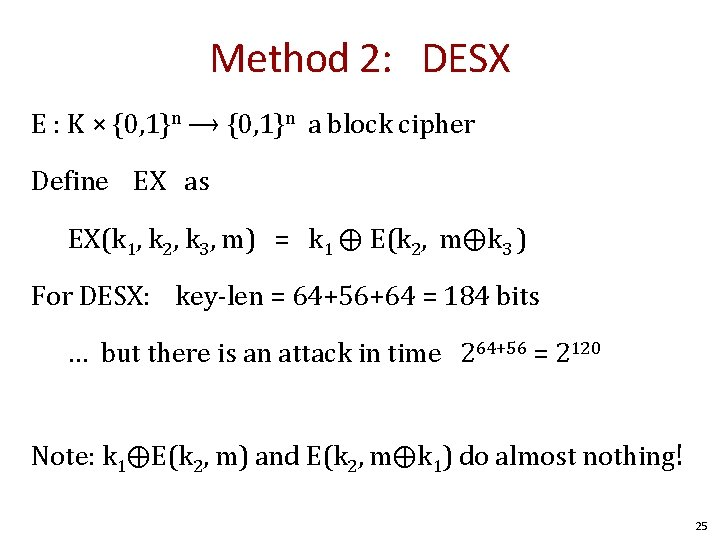Method 2: DESX E : K × {0, 1}n ⟶ {0, 1}n a block cipher Define EX as EX(k 1, k 2, k 3, m) = k 1 ⨁ E(k 2, m⨁k 3 ) For DESX: key-len = 64+56+64 = 184 bits … but there is an attack in time 264+56 = 2120 Note: k 1⨁E(k 2, m) and E(k 2, m⨁k 1) do almost nothing! 25Attacks on the implementation 1. Side channel attacks: – Measure time to do enc/dec, measure power for enc/dec 16 rounds [Kocher, Jaffe, Jun, 1998] Card is doing DES smartcard IP IP-1 2. Fault attacks: – Computing errors in the last round expose the secret key k ⇒ never implement crypto primitives yourself … 26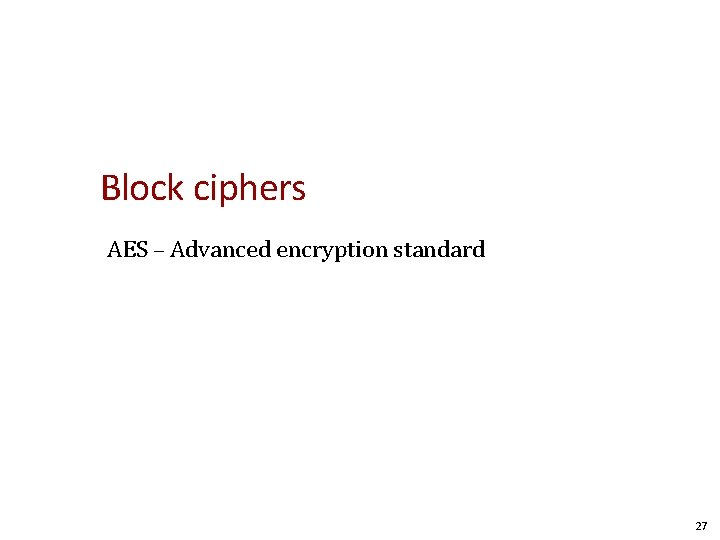Block ciphers AES – Advanced encryption standard 27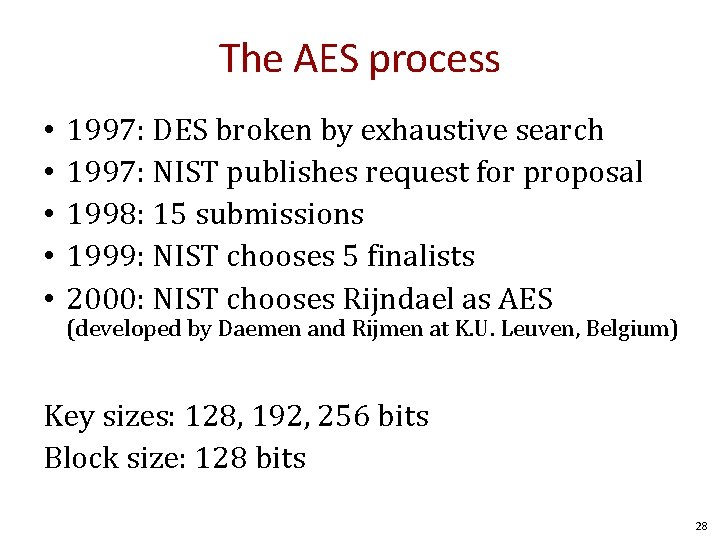The AES process • • • 1997: DES broken by exhaustive search 1997: NIST publishes request for proposal 1998: 15 submissions 1999: NIST chooses 5 finalists 2000: NIST chooses Rijndael as AES (developed by Daemen and Rijmen at K. U. Leuven, Belgium) Key sizes: 128, 192, 256 bits Block size: 128 bits 28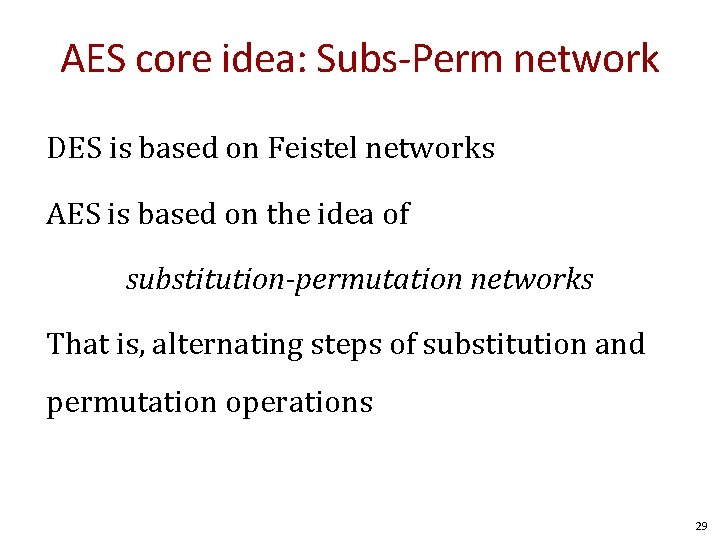AES core idea: Subs-Perm network DES is based on Feistel networks AES is based on the idea of substitution-permutation networks That is, alternating steps of substitution and permutation operations 29Modes of operation How to encrypt messages longer than a block size. 30Recall: Semantic security under CPA Modes that return the same ciphertext (e. g. , ECB) for the same plaintext are not semantically secure under a chosen plaintext attack (CPA) (many-time-key) Two solutions: 1. Randomized encryption 2. Stateful (Nonce-based) encryption 31Nonce-based encryption Nonce n: a value that changes for each msg. E(k, m, n) / D(k, c, n) m, n E(k, m, n) = c, n E c, n D(k, c, n) = m D k k (k, n) pair never used more than once 32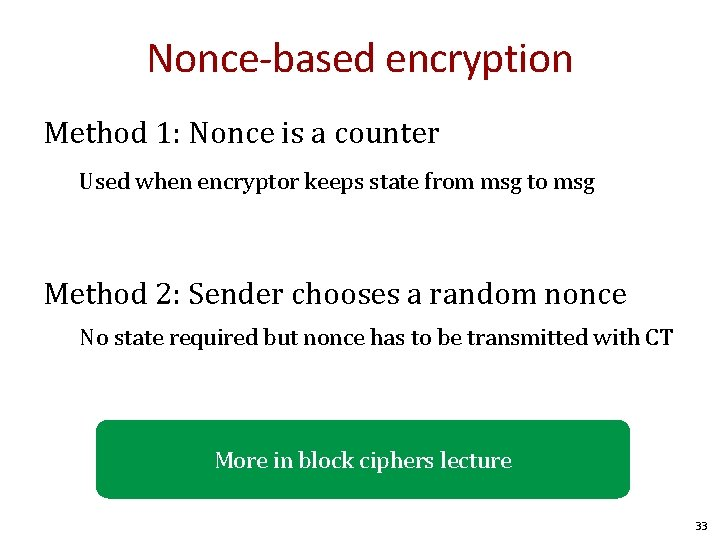Nonce-based encryption Method 1: Nonce is a counter Used when encryptor keeps state from msg to msg Method 2: Sender chooses a random nonce No state required but nonce has to be transmitted with CT More in block ciphers lecture 33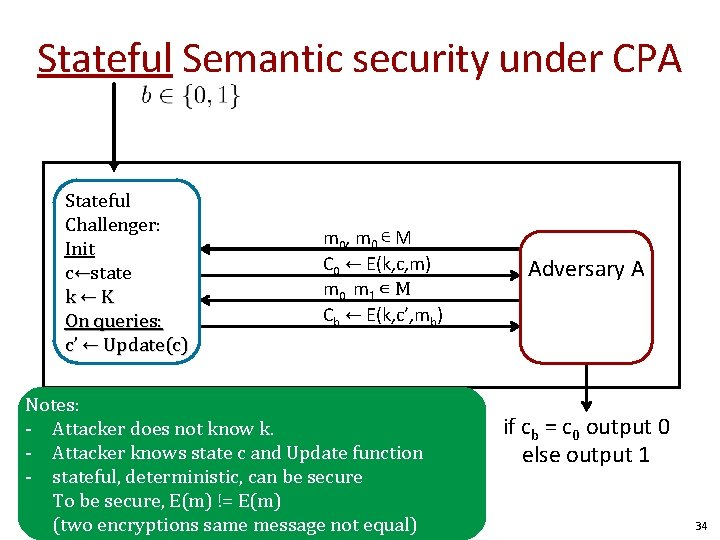Stateful Semantic security under CPA Stateful Challenger: Init c←state k←K On queries: c’ ← Update(c) m 0 , m 0 ∊ M C 0 ← E(k, c, m) m 0, m 1 ∊ M Cb ← E(k, c’, mb) Notes: - Attacker does not know k. - Attacker knows state c and Update function - stateful, deterministic, can be secure To be secure, E(m) != E(m) (two encryptions same message not equal) Adversary A if cb = c 0 output 0 else output 1 34Stateless Semantic security under CPA Stateless Challenger: Init c←rand k←K On queries: c’ ← rand m 0 , m 0 ∊ M C 0 ← E(k, c, m) m 0, m 1 ∊ M Cb ← E(k, c’, mb) Notes: - Attacker does not know k. - Attacker does not know c - To be secure, E(m) != E(m) (two encryptions same message not equal) Adversary A if cb = c 0 output 0 else output 1 35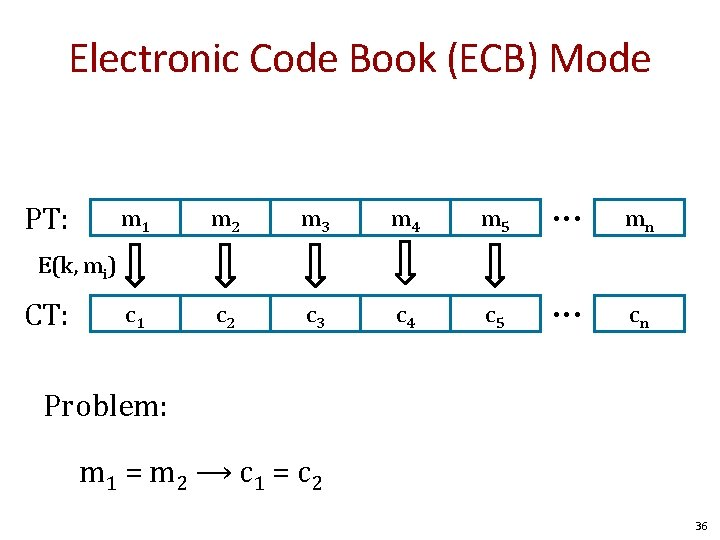Electronic Code Book (ECB) Mode PT: m 1 m 2 m 3 m 4 m 5 • • • mn c 1 c 2 c 3 c 4 c 5 • • • cn E(k, mi) CT: Problem: m 1 = m 2 ⟶ c 1 = c 2 36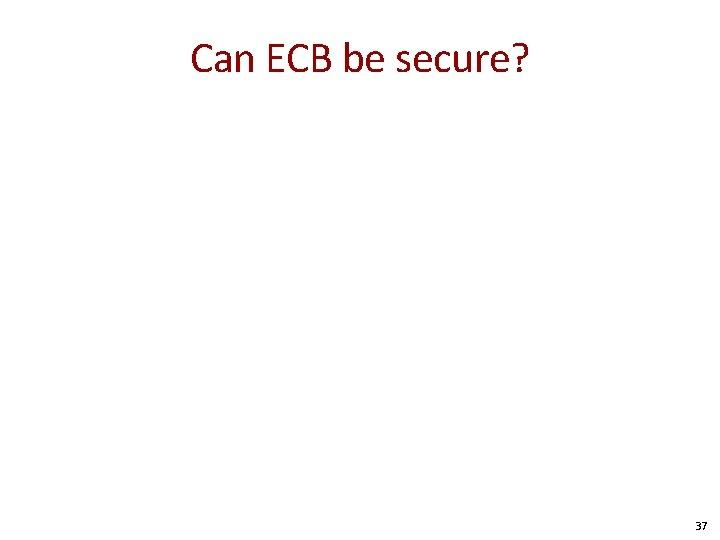Can ECB be secure? 37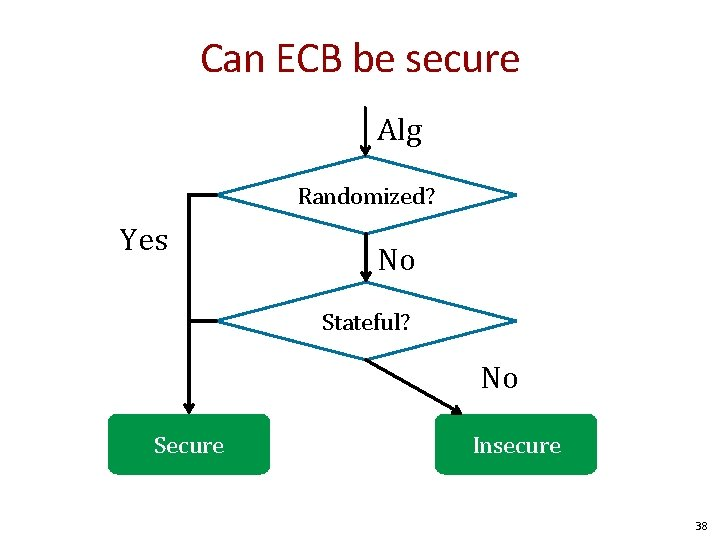Can ECB be secure Alg Randomized? Yes No Stateful? No Secure Insecure 38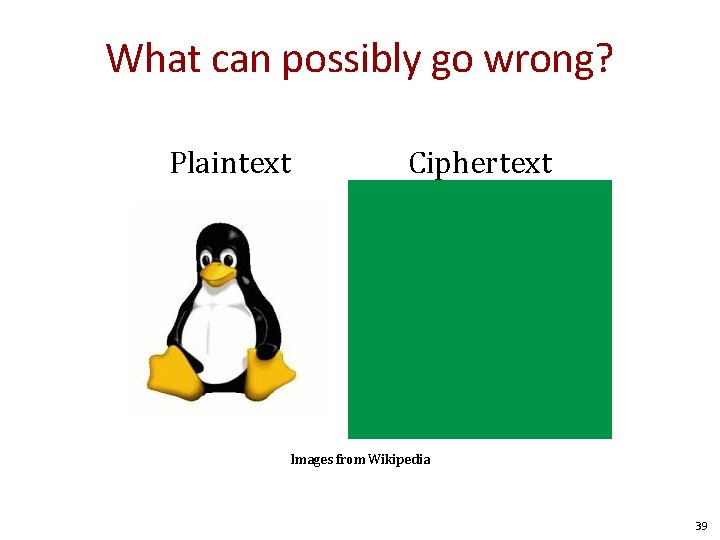What can possibly go wrong? Plaintext Ciphertext Images from Wikipedia 39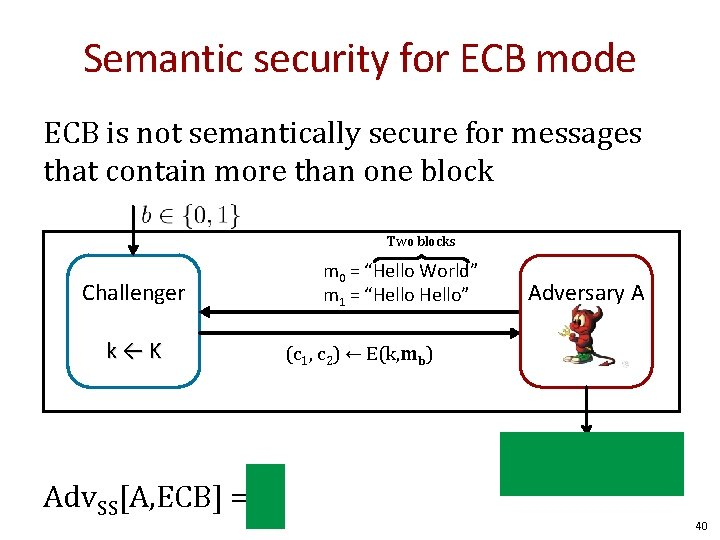Semantic security for ECB mode ECB is not semantically secure for messages that contain more than one block Two blocks Challenger k←K Adv. SS[A, ECB] = 1 m 0 = “Hello World” m 1 = “Hello” Adversary A (c 1, c 2) ← E(k, mb) if c 1 = c 2 output 1 else output 0 40Stateful Counter Mode • Parallel encryption/stream encryption • Allows construction of a stream cipher built from a PRF/PRP F (e. g. AES, 3 DES) • Better than ECB but only works as long as the key is only used once (one-time-key) 41Stateful Counter Mode is Secure Theorem: For any L > 0, If F is a secure PRF over (K, X, X) then EDETCTR is a sem. secure cipher over (K, XL). In particular, for any eff. adversary A attacking EDETCTR there exists an eff. PRF adversary B s. t. : Adv. SS[A, EDETCTR] = 2 ∙Adv. PRF[B, F] 42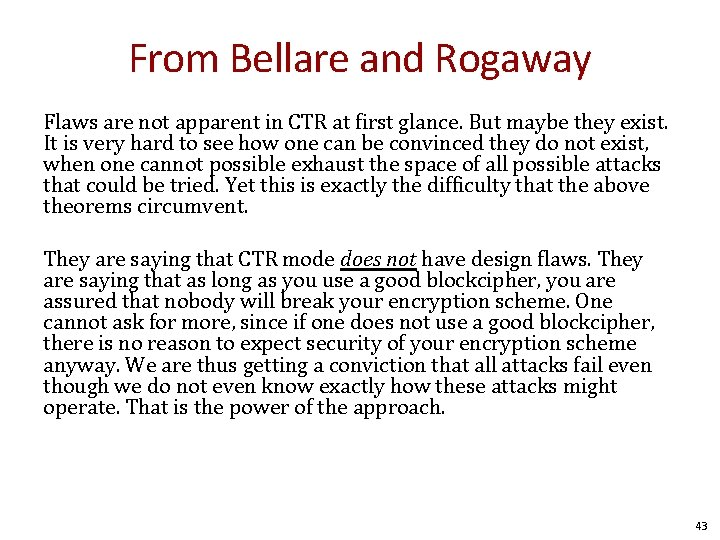From Bellare and Rogaway Flaws are not apparent in CTR at first glance. But maybe they exist. It is very hard to see how one can be convinced they do not exist, when one cannot possible exhaust the space of all possible attacks that could be tried. Yet this is exactly the difficulty that the above theorems circumvent. They are saying that CTR mode does not have design flaws. They are saying that as long as you use a good blockcipher, you are assured that nobody will break your encryption scheme. One cannot ask for more, since if one does not use a good blockcipher, there is no reason to expect security of your encryption scheme anyway. We are thus getting a conviction that all attacks fail even though we do not even know exactly how these attacks might operate. That is the power of the approach. 43Secret in model Stateless Counter Mode 44Cipher block chaining mode (CBC) Let(E, D) be a PRP. ECBC(k, m): choose random IV ∊ X and do: IV IV ciphertext m m m m ⊕ ⊕ E(k, ∙) c c c c Decryption: c = E(k, IV⊕m) ⟶ m = D(k, c) ⊕ IV 45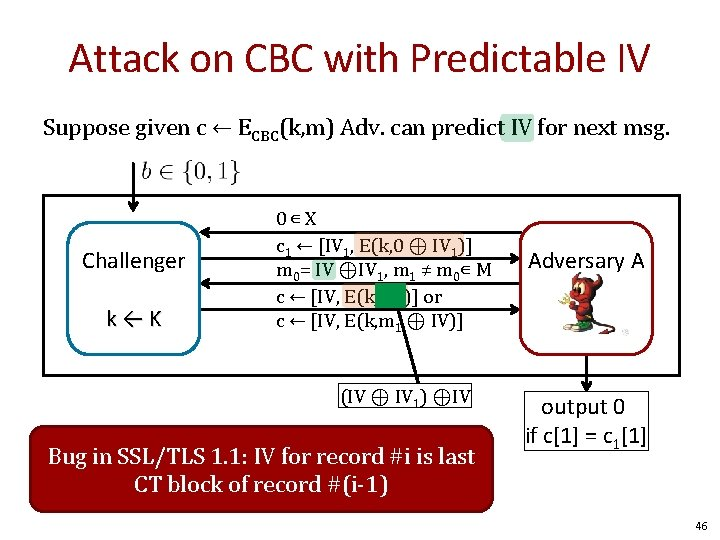Attack on CBC with Predictable IV Suppose given c ← ECBC(k, m) Adv. can predict IV for next msg. Challenger k←K 0∊ X c 1 ← [IV 1, E(k, 0 ⊕ IV 1)] m 0= IV ⊕IV 1, m 1 ≠ m 0∊ M c ← [IV, E(k, IV 1)] or c ← [IV, E(k, m 1 ⊕ IV)] (IV ⊕ IV 1) ⊕IV Bug in SSL/TLS 1. 1: IV for record #i is last CT block of record #(i-1) Adversary A output 0 if c = c 1 46CBC: padding nonce m m m ⊕ ⊕ E(k, ∙) E(k, ∙) nonce c c c c IV TLS: for n > 0 n byte pad is: n n … n If no pad needed, add a dummy block: 16 16 m || pad removed during decryption … 16 Padding oracle side channel attacks 47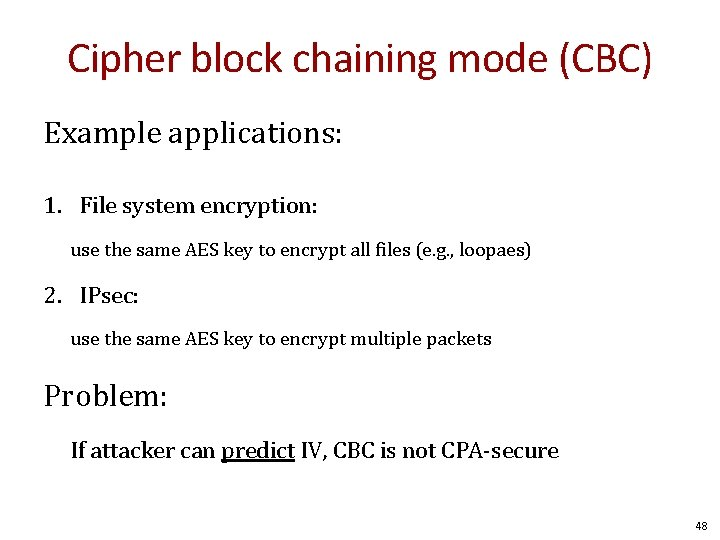Cipher block chaining mode (CBC) Example applications: 1. File system encryption: use the same AES key to encrypt all files (e. g. , loopaes) 2. IPsec: use the same AES key to encrypt multiple packets Problem: If attacker can predict IV, CBC is not CPA-secure 48A Simplified Example (Motivated from TLS) type||ver||len data <mac> pad Assume block cipher is 64 -bits – Any message not a multiple of 8 bytes is padded Valid pad: – 1 byte needed: 0 x 1 – 2 bytes needed: 0 x 2 –. . – No padding: 0 x 8 0 x 8 49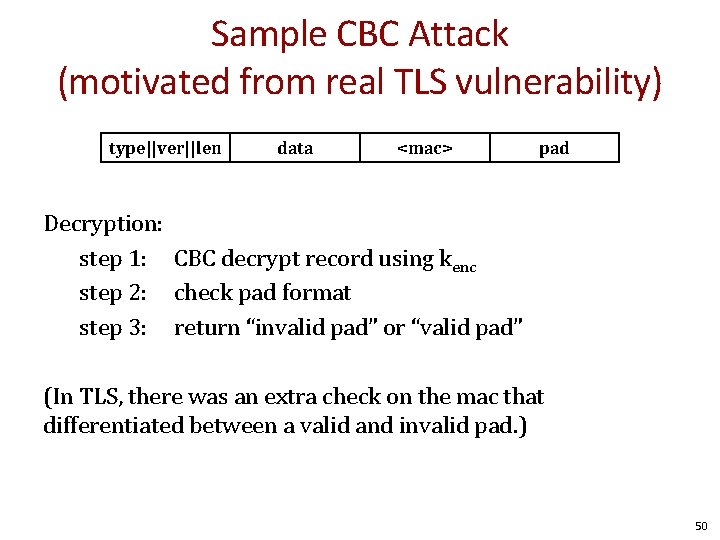Sample CBC Attack (motivated from real TLS vulnerability) type||ver||len data <mac> pad Decryption: step 1: CBC decrypt record using kenc step 2: check pad format step 3: return “invalid pad” or “valid pad” (In TLS, there was an extra check on the mac that differentiated between a valid and invalid pad. ) 50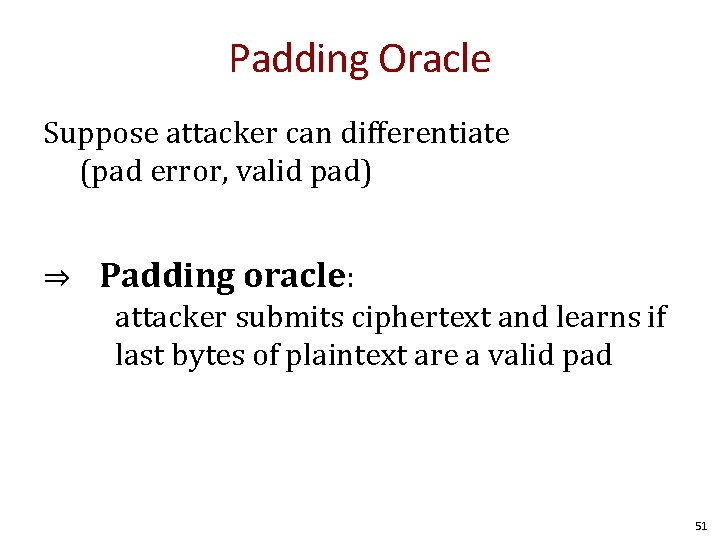Padding Oracle Suppose attacker can differentiate (pad error, valid pad) ⇒ Padding oracle: attacker submits ciphertext and learns if last bytes of plaintext are a valid pad 51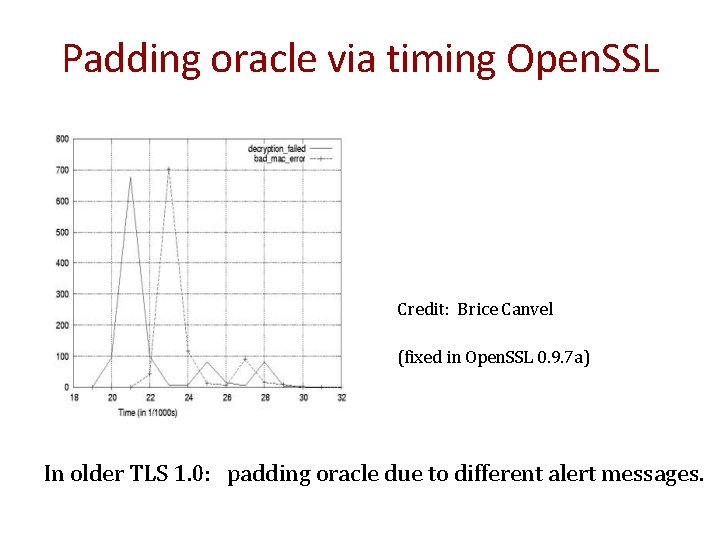Padding oracle via timing Open. SSL Credit: Brice Canvel (fixed in Open. SSL 0. 9. 7 a) In older TLS 1. 0: padding oracle due to different alert messages.Summary Block ciphers – Map fixed length input blocks to same length output blocks – Canonical block ciphers: 3 DES, AES – Block cipher modes – CBC attacks – Never return an error that is informative. 53Questions? 54END